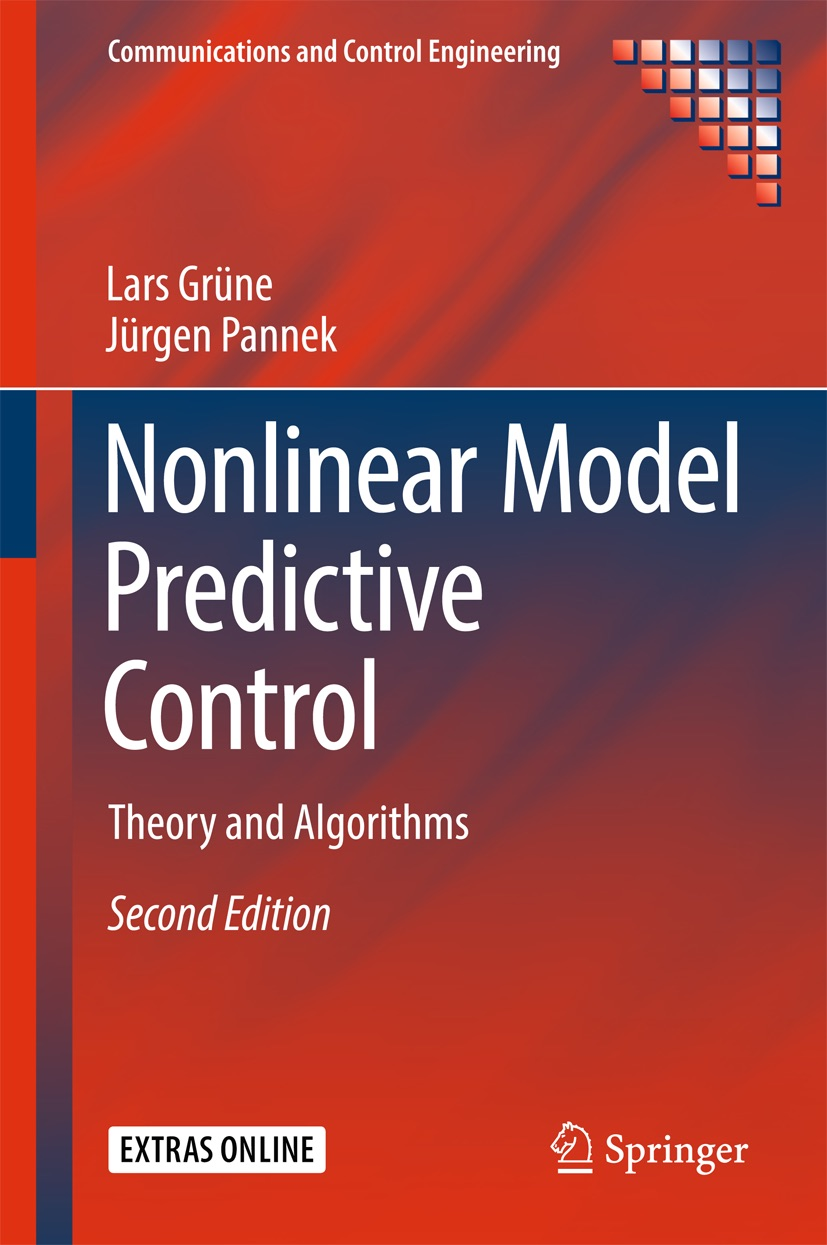## MATLAB NMPC routine and examples

All of the "simpler" examples in the book were computed with our MATLAB NMPC routine "nmpc.m". Here you can download this routine and the M-Files for the individual examples which use this routine. The code should work for MATLAB releases R2007a and newer and requires the optimization toolbox. For more information see Section A.1 in the book and the comments and help texts in each file.

MATLAB NMPC routine: nmpc.m

(Version of 30th May 2011 in which a bug in the constraint handling has been fixed)

M-Files for examples in the book using the MATLAB NMPC routine "nmpc.m":

Example 6.31 (6.26 in 1st ed.): example_6_26.m

Example 6.38 (6.34 in 1st ed.): example_6_34.m

Example 7.12 (8.12 in 1st ed.): example_8_12.m

Example 7.31 (8.31 in 1st ed.): example_8_31.m

Example 7.39 (8.39 in 1st ed.): example_8_39.m

Example 8.1: example_8_1.m

Example 8.16: example_8_16.m

Example 8.23: example_8_23.m

Example 8.28: example_8_28.m

Example 8.34: example_8_34.m

Example 8.40(i): example_8_40i.m

Example 8.40(ii): example_8_40ii.m

Example 8.40(iii): example_8_40iii.m

Example 10.2 (7.2 in 1st ed.): example_7_2.m

The following file contains the minimal amount of declarations needed in order to call the NMPC routine "nmpc.m". It can be used as a template for own examples.

Minimal example: minimal_example.m

ZIP archive with NMPC routine and minimal example: matlab_nmpc.zip

ZIP archive with all examples for the 1st edition: matlab_nmpc_exam_1ed_6_7_8.zip
complete ZIP archive for the 1st edition: matlab_nmpc_w_exam_1ed.zip

ZIP archive with all examples for the 2nd edition: matlab_nmpc_exam_2ed_8.zip
complete ZIP archive for the 2nd edition: matlab_nmpc_w_exam_2ed.zip

→ back to homepage www.nmpc-book.com

© 2010-2016 Lars Grüne, Jürgen Pannek